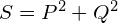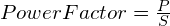# Active, Reactive & Apparent Power | Easiest Explanation

Active, Reactive & Apparent Power | Easiest Explanation 1024 576 Gaurav J

Knowledge of active, reactive and apparent power is a must for an Electrical Engineer. But most of the time we end up with confusion in all these powers. And hence, if you want to have the explanation of active, reactive and apparent power crystal clear then I would recommend you to watch this tutorial.

##### In this tutorial we’ll learn about

In the end of this tutorial we will also have information about power factor so, make sure you read it till the end. Before we start with the explanation, please note, the concept of active, reactive and apparent power is applicable only for AC systems. Concept of Active, reactive and apparent powers are not applicable for DC systems.
To understand what is active, reactive and apparent power we first must know what is instantaneous power.

## Instantaneous power

To understand the instantaneous power consider following example. A resistive load is connected to a 230V AC supply.

Now let’s say, I want to calculate power at an instant “t”, and to do that I need to multiply voltage and current at the instant “t”. That will give us the power at a particular instant “t”. This power is called as instantaneous power. Why instantaneous? Because we measured it at a particular instant.

This instantaneous power can be positive or negative. Now, you can ask what is a positive power or negative power? So, let’s understand the concept of positive power and negative power.

#### Positive Power

Power is called as positive power when it flows from Source to load. In our above example, power is positive, if it is flowing from 230 V AC supply to load.

#### Negative Power

When power flows from lord to source, that power is called as negative power. In our above example, power is negative, if it is flowing from load to 230 V AC supply.

Now, the question is how can power flow from load to source? And in which case this happens? We will see about this in a few minutes.

Go to content

## Active Power (P)

To understand active power, again consider the circuit shown below. In the below circuit, we have connected a 230 V AC supply to a purely resistive load.

As we know, in purely resistive Circuit voltage and current are in phase. In phase means,

• voltage and current reaches their positive peak at the same time
• They become zero at the same time
• Also they reach their negative peak at the same time.

If you draw voltage and current waveform of a resistive circuit it will look like this.

To calculate power in this circuit you can multiply voltage and current at any instant, and you will find that the resultant power is only positive power.

And such power which remains positive always is called as active power.

### Properties of active power

1. It is always positive
2. It does not changes its direction
3. Power flow is always from source to load
4. Denoted by letter “P” and measured in Watts

Go to content

## Reactive Power (Q)

To understand what is reactive power, we will replace the resistive load with purely capacity load in our example, as shown in below figure.

If you draw voltage and current view form for this circuit it will look like this.

As you can see, current has a head start than the voltage. Or simply, current is leading the voltage. This indicates that, voltage and current are out of phase in this circuit. Out of phase means,

• Voltage and current do not reach their positive peak at the same time
• They do not become zero at the same time
• And they also do not reach their negative peak at the same time.

So, if you calculate power at the instant shown in the below figure, you will get a positive power because both voltage and current are positive.

If you calculate power at the instant shown below, you will get a negative power because, voltage is positive but the current is negative. And negative multiply by positive is Negative.

What does this negative power indicate? This tells us that, power is flowing from load to source.
If you continue calculating power in the circuit the waveform will continue.

This power is going forward and coming backward like a pendulum without doing any useful work in the system. And this type of power is called as reactive power.

Capacitor, inductor and any non liner device can inject/absorb the Reactive power into the system.

#### Why Power flows from load to source?

When power is in positive, capacitor charges or its stores energy in it. When power becomes negative, capacitor discharges or releases the stored energy. And this is the reason why power flows from load to source.

### Properties of reactive power

1. This power can be positive as well as negative.
2. It only represent the power which goes back and forth without doing any useful work.
3. Denoted by letter “Q” and measured in VAR (Volt Ampere Reactive).
4. Capacitor, inductor and any non liner device can inject/absorb the Reactive power into the system

## Distinction between active and reactive power

1. We cannot convert active power into reactive, and reactive power into active.
2. Active power is a separate quantity and reactive power is a separate quantity.
3. Both the power place a burden on the transmission line.
4. Active power produce heat, mechanical power, light etc.
5. Reactive power only represent power that oscillates back and forth.

You can also watch the detailed tutorial on Difference between active and reactive power.

Go to content.

## Apparent power (S)

In a system, you will have all the types of loads at the same time. You can have resistive load, you can also have inductive load or capacitive load, or maybe the combination of all types of loads. Consider the below example, in which we have resistive load and an inductive load connected to the same source.

Resistive load will consume active power and inductive load will consume reactive power. Now, we cannot say that the circuit is consuming active power or reactive power because it is consuming both powers. And hence, we need a different name for the combination of active and reactive power. So, this type of combination of both the powers is called as Apparent power.

The combination of active power and reactive power is called as apparent power.

We can calculate apparent power by,Apparent power is denoted by letter “S” and it is measured in VA/KVA/MVA. Transformers are rated in VA/KVA/MVA.

Goto the content.

## Power Factor

Power factor is very closely related to active, reactive and apparent power and hence I am briefing it here. If you would like to know in detail about power factor I have a separate playlist on that which you can watch here.

If you ask any electrical engineer to define Power factor, he/she will say, ‘power factor is the angle between voltage and current’. This may be the true definition but it is not the proper way of defining power factor.
Proper definition of power factor is,

“The ratio of Active power to the Apparent power is called as power factor”.When, someone says, power factor of the system is 0.8, what does that mean? This simply means that, out for 100% power, 80% is active power and reaming 20% is reactive power.

Power factor tells about how much active power a system/equipment is consuming.

Goto the content.###### Gaurav J

Electrical Engineer. Content Creator. Currently working with a High & Extra High Voltage Switchgear Industry.

All stories by: Gaurav J